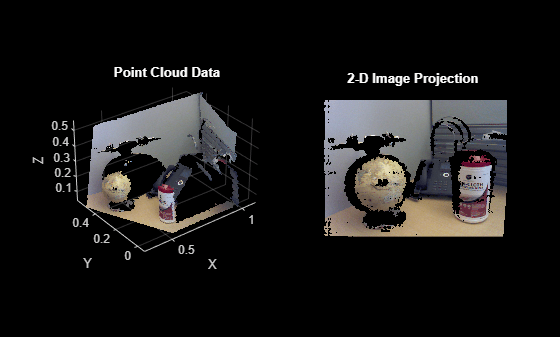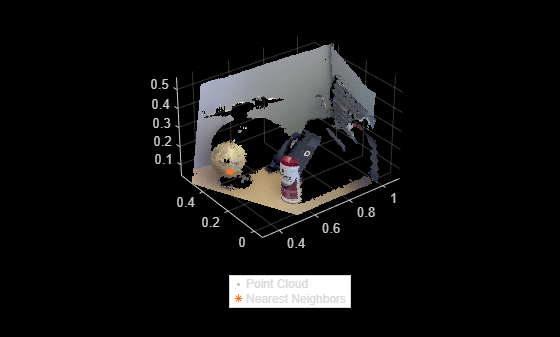# estimateCameraProjection

Estimate camera projection matrix from world-to-image point correspondences

## Syntax

``camProjection = estimateCameraProjection(imagePoints,worldPoints)``
``[camProjection,reprojectionErrors] = estimateCameraProjection(imagePoints,worldPoints)``

## Description

````camProjection = estimateCameraProjection(imagePoints,worldPoints)` returns the camera projection matrix determined from known world points and their corresponding image projections by using the direct linear transformation (DLT) approach.```

example

````[camProjection,reprojectionErrors] = estimateCameraProjection(imagePoints,worldPoints)` also returns the reprojection error that quantifies the accuracy of the projected image coordinates.```

## Examples

collapse all

Load a 3-D point cloud data captured by an RGB-D sensor into the workspace.

`load("object3d.mat");`

Remove points with `Inf` or `NaN` coordinates from the point cloud.

`[validPtCloud,validIndices] = removeInvalidPoints(ptCloud);`

Read the valid world point coordinates. Each entry specifies the (x, y, z) coordinates of a point in the point cloud.

`worldPoints = validPtCloud.Location;`

Define the corresponding image point coordinates as a orthographic projection of point cloud data onto the yz-plane.

```indices = 1:ptCloud.Count; [y,z] = ind2sub([size(ptCloud.Location,1),size(ptCloud.Location,2)],indices); imagePoints = [y(validIndices)' z(validIndices)'];```

Generate the 2-D image projection by using the image point coordinates and their color values.

```projImage = zeros(max(imagePoints(:,1)),max(imagePoints(:,2)),3); rgb = validPtCloud.Color; for j = 1:length(rgb) projImage(imagePoints(j,1),imagePoints(j,2),:) = rgb(j,:); end```

Display the point cloud data and the corresponding 2-D image projection.

```figure subplot(1,2,1) pcshow(ptCloud) xlabel("X"); ylabel("Y"); zlabel("Z"); title("Point Cloud Data",Color=[1 1 1]) subplot(1,2,2) imshow(uint8(projImage)) title("2-D Image Projection",Color=[1 1 1])```Estimate the camera projection matrix and reprojection error by using the known world points and the image points.

`[camProjection,reprojectionErrors] = estimateCameraProjection(imagePoints,worldPoints);`

Use the estimated camera projection matrix as input to the `findNearestNeighbors` function and find the nearest neighbors of a query point.

```point = [0.4 0.3 0.2]; K = 50; % Specify the number of nearest neighbors to be determined [indices,dists] = findNearestNeighbors(ptCloud,point,K,camProjection');```

Use the `select` function to get the point cloud data of nearest neighbors.

`ptCloudB = select(ptCloud,indices); `

Display the input point cloud and its nearest neighbors.

```figure pcshow(ptCloud) hold on plot3(ptCloudB.Location(:,1),ptCloudB.Location(:,2),ptCloudB.Location(:,3),"*") hold off legend("Point Cloud","Nearest Neighbors",Location="southoutside",Color=[1 1 1])```## Input Arguments

collapse all

Coordinates of image projection points, specified as an M-by-2 matrix of (x, y) coordinates. M is the number of points and it must be greater than or equal to 6. The input image points must correspond to an undistorted image plane.

Data Types: `single` | `double`

3-D world points, specified as an M-by-3 matrix of non-coplanar (x, y, z) coordinates. M is the number of points and must be greater than or equal to 6.

Data Types: `single` | `double`

## Output Arguments

collapse all

Camera projection matrix, returned as a 3-by-4 matrix. The matrix maps the 3-D world points, in homogenous coordinates, to the 2-D image coordinates of the projections onto the image plane.

Using the camera projection matrix and homogeneous coordinates, you can project a world point onto the image.

w × [x,y,1]' = `camProjection`× [X,Y,Z,1]'

 (X,Y,Z) are the world coordinates of a point (x,y) are the coordinates of the corresponding image point w is an arbitrary scale factor

Data Types: `double`

Reprojection errors, returned as a M-by-1 vector. The reprojection error is the error between the reprojected image points and the input image points. For more information on the computation of reprojection errors, see Algorithms.

Data Types: `double`

## Algorithms

Given the world points X and the image points x, the camera projection matrix C, is obtained by solving the equation

λx = CX.

The equation is solved using the direct linear transformation (DLT) approach . This approach formulates a homogeneous linear system of equations, and the solution is obtained through generalized eigenvalue decomposition.

Because the image point coordinates are given in pixel values, the approach for computing the camera projection matrix is sensitive to numerical errors. To avoid numerical errors, the input image point coordinates are normalized, so that their centroid is at the origin. Also, the root mean squared distance of the image points from the origin is `sqrt(2)`. These steps summarize the process for estimating the camera projection matrix.

1. Normalize the input image point coordinates with transform T.

2. Estimate camera projection matrix CN from the normalized input image points.

3. Compute the denormalized camera projection matrix C as CNT-1.

4. Compute the reprojected image point coordinates xE as CX.

5. Compute the reprojection errors as

reprojectionErrors = |xxE|.

 Hartley, Richard, and Andrew Zisserman. Multiple View Geometry in Computer Vision. 2nd ed. Cambridge, UK ; New York: Cambridge University Press, 2003.